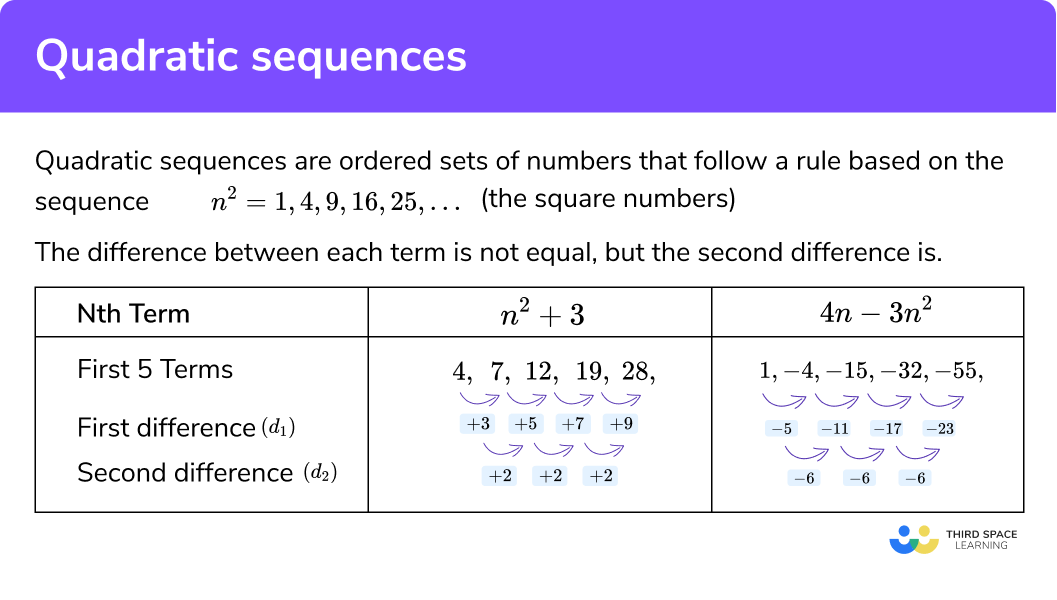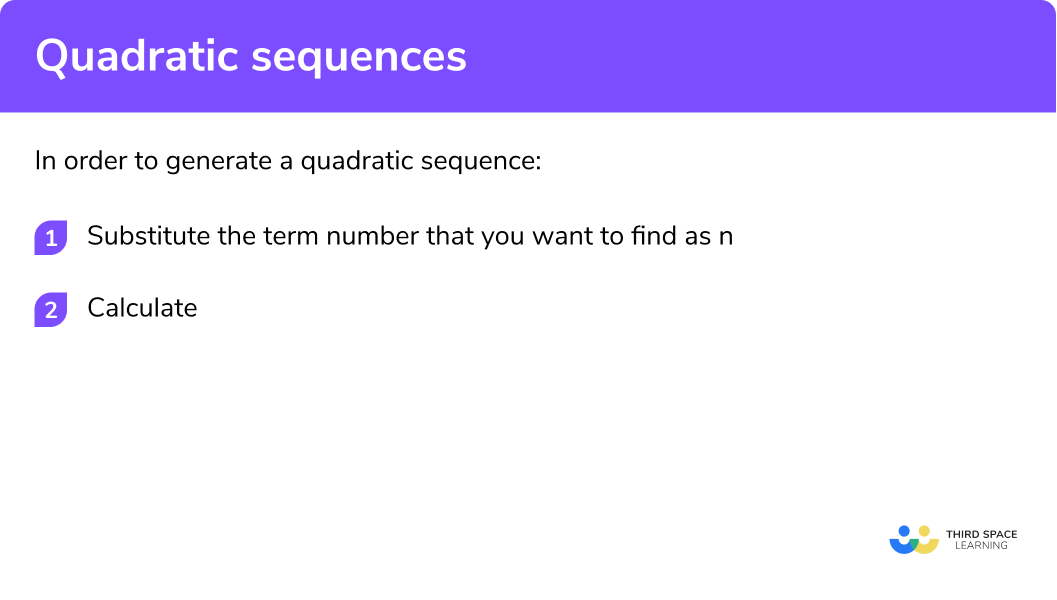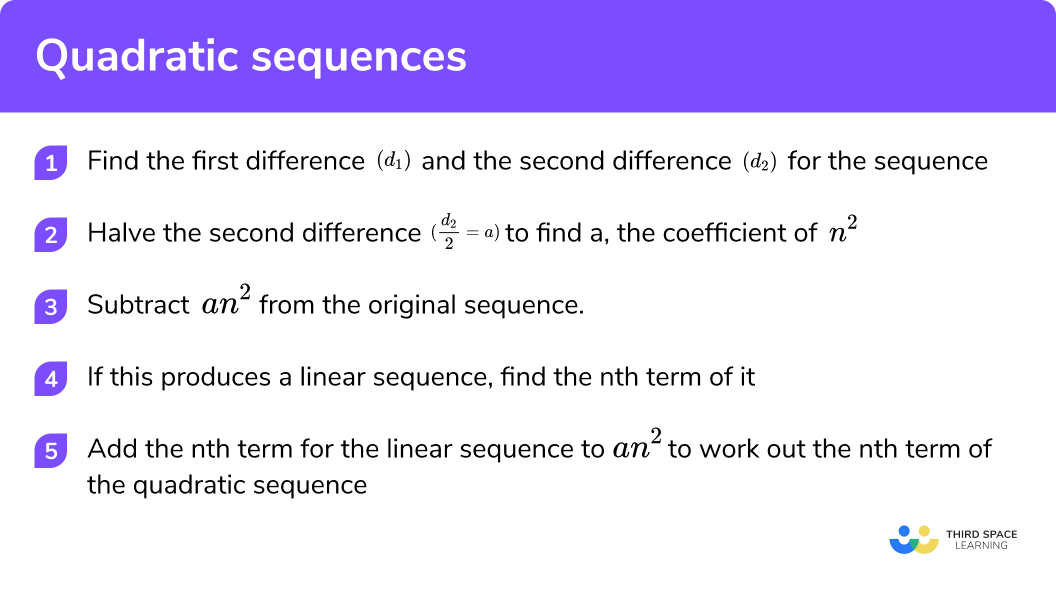GCSE Maths Algebra Sequences

Here we will learn about quadratic sequences including how to recognise, use and find the nth term of a quadratic sequence.

There are also quadratic sequences worksheets based on Edexcel, AQA and OCR exam questions, along with further guidance on where to go next if you’re still stuck

Quadratic sequences are only studied on the higher GCSE Maths pathway.

Quadratic sequences are ordered sets of numbers that follow a rule based on the sequence n2 = 1, 4, 9, 16, 25,… (the square numbers).

Quadratic sequences always include an n2 term.

The difference between each term in a quadratic sequence is not equal, but the second difference between each term in a quadratic sequence is equal.

Here are two examples of quadratic sequences:
4, 7, 12, 19, 28 requires adding to work out that the second difference is +2
and
1, −4, −15, −32, −55 requires subtracting to work out that the second difference is −6

Example:## How to generate terms of quadratic sequences

1. Substitute the term number that you want to find as n.
2. Calculate.

### Explain how to generate quadratic sequences in 2 steps### Related lessons on sequences

Quadratic sequences is part of our series of lessons to support revision on sequences. You may find it helpful to start with the main sequences lesson for a summary of what to expect, or use the step by step guides below for further detail on individual topics. Other lessons in this series include:

The quadratic sequence formula is: an^{2}+bn+c

Where,

a, b and c are constants (numbers on their own)

n is the term position

We can use the quadratic sequence formula by looking at the general case below:

Let’s use this to work out the n^{th} term of the quadratic sequence,

4, 5, 8, 13, 20, ...

First let’s find the first and second differences of the sequence:

Comparing this to the general case above, we can see that:

The second difference is equal to 2 so,

The first difference is equal to 1 so,

The first term is equal to 4 so,

We can see that a = 1,\; b = -2 and c = 5

So the nth of this quadratic sequence using the quadratic sequences formula is:

n^{2}-2n+5

## Generate terms of quadratic sequences examples

### Example 1: finding terms of a sequence

Find the first three terms of the sequence with nth term 2n2 + 4n + 1.

1. Substitute the term number that you want to find as n.

We want to find terms 1, 2 and 3 so substituting each of these into the formula
2n2 + 4n + 1 we get,

First term:
n = 1:   (2 × 12 ) + (4 × 1) + 1

Second term:
n = 2:   (2 × 22 ) + (4 × 2) + 1

Third term:
n = 3:   (2 × 32 ) + (4 × 3) + 1

2 Calculate.

Remember to apply BIDMAS

n = 1:   (2 × 12 ) + (4 × 1) + 1 = 7

n = 2:   (2 × 22 ) + (4 × 2) + 1 = 17

n = 3:   (2 × 32 ) + (4 × 3) + 1 = 31

The first term is 7, the next term is 13 and the term after that is 31.

### Example 2: finding terms of a sequence

Find the tenth term of the sequence with nth term 5n2 − 6n − 3.

We want to find term 10 so we need to substitute this into the formula

5n2 − 6n − 3.

$(5 \times 10^{2}) − (6 \times 10) − 3$
$(5 \times 10^{2}) − (6 \times 10) − 3 = 437$

The tenth term is 437.

## How to find the nth term of quadratic sequences

In order to find the nth term (general term) of a quadratic sequence we have to find the second difference. To do this, we calculate the first difference between each term in a quadratic sequence and then calculate the difference between this new sequence.

1 Find the first difference (d1) and second difference (d2) for the sequence.

2 Halve the second difference

$\left ( \frac{d_{2}}{2} =a \right )$

to find a, the coefficient of n2.

3 Subtract an2 from the original sequence.

4 If this produces a linear sequence, find the nth term of it.

5 Add the nth term for the linear sequence to an2 to work out the nth term of the quadratic sequence.

### What are the 5 steps to find the nth term of a sequence?Step 4 is an additional step dependent on whether there is a remainder in step 3.

• If there is no remainder after subtracting an2 from the original sequence, step 4 is not needed.
• If the same constant remains for each term after the subtraction, we just add it to an2.
• If a sequence is generated after step 3, continue with step 4.

See the table below.

Here,

• Sequence 1 had no remainder and so the sequence is 2n2
• Sequence 2 had a remainder of 1 and so this sequence is 2n2 + 1
• Sequence 3 had another sequence as the remainder and so the nth term of this linear sequence was calculated and added to 2n2 to get 2n2 + n + 2.

## Find the nth term of quadratic sequences examples

### Example 3: find the nth term of a quadratic sequence of the form an2.

Find the nth term of this quadratic sequence:

2, 8, 18, 32, 50,

Here, the second difference d2 = 4.

$\left ( \frac{d_{2}}{2} =a \right )$

to find a, the coefficient of n2.

d2 = 4,

$4 \div 2 = 2$

This means a = 2 and so we have the sequence 2n2.

Here, the remainder for each term is 0. This means that we have found the nth term of the quadratic sequence.

Not required for this example as the remainder is 0 for each term.

The nth term of the quadratic sequence is 2n2.

### Example 4: find the nth term of a quadratic sequence of the form an2 + c.

Find the nth term rule of the quadratic sequence:

5, 14, 29, 50, 77,

Here, the second difference d2 = 6.

$\left ( \frac{d_{2}}{2} =a \right )$

to find a, the coefficient of n2.

d2 = 6,

$6 \div 2 = 3$

This means a = 3 and so we have the sequence 3n2.

Here, the remainder for each term is 2.

Not required for this example as the remainder is 2 for each term.

The nth term of the quadratic sequence is 3n2 + 2.

### Example 5: find the nth term of a quadratic sequence of the form an2 + bn + c.

Find the nth term of this quadratic sequence:

9, 18, 31, 48, 69, …

Here, the second difference d2 = 4.

$\left ( \frac{d_{2}}{2} =a \right )$

to find a, the coefficient of n2.

d2 = 4,

$4 \div 2 = 2$

This means a = 2 and so we have the sequence 2n2.

Here, the remainder generates a linear sequence and so we must find the nth term for this sequence as well.

Here, the nth term for the remainder is 3n + 4.

Here, the nth term of the quadratic sequence is 2n2 + 3n + 4.

### Example 6: find the nth term of a quadratic sequence of the form an2 + bn + c.

Find the nth term of this quadratic sequence:

19, 36, 61, 94, 135,

Here, the second difference d2 = 8.

$\left ( \frac{d_{2}}{2} =a \right )$

to find a, the coefficient of n2.

d2 = 8,

$8 \div 2 = 4$

This means a = 4 and so we have the sequence 4n2.

Here, the remainder generates a linear sequence and so we must find the nth term for this sequence as well.

Here, the nth term for the remainder is 5n + 10.

Here, the nth term of the quadratic sequence is 4n2 + 5n + 10.

### Example 7: find the nth term of a quadratic sequence of the form an2 + bn + c where terms are generated using a pattern.

In the diagram below, the area of each pool can be represented by a quadratic sequence.

Find the area for a pool of width n.

Here, the second difference d2 = 2.

$\left ( \frac{d_{2}}{2} =a \right )$

to find a, the coefficient of n2.

d2 = 2,

$2 \div 2 = 1$

This means a = 1 and so we have the sequence n2.

Here, the remainder for each term is 0. This means that we have found the nth term of the quadratic sequence.

Not required for this example as the remainder is 0 for each term.

The nth term of the quadratic sequence is n2.

So the area of the pool with width n would be n2.

### Example 8: find the nth term of a quadratic sequence of the form an2 + bn + c where a < 0.

Find the nth term of this quadratic sequence:

8, -10, -34, -64, -100,

Here, the second difference d2 = −6.

$\left ( \frac{d_{2}}{2} =a \right )$

to find a, the coefficient of n2.

d2 = −6,

$−6 \div 2 = −3$

This means a = −3 and so we have the sequence −3n2.

Here, the remainder generates a linear sequence and so we must find the nth term for this sequence as well.

Here, the nth term of the quadratic sequence is −3n2 − 9n + 20.

### Common misconceptions

• The value of the second difference is not halved before using it as the coefficient of n2

For example, for the quadratic sequence n2 = 1, 4, 9, 16, 25, … the second difference is equal to 2 and so the nth term is incorrectly written as 2n2.

• The quadratic sequence is answered as if it were an arithmetic sequence

For a quadratic sequence we will have a common second difference.

• When the sequence is decreasing into negative terms, the second difference is written incorrectly as a positive number

For example, the sequence −n2 = −1, −4, −9, −16, … has a second difference of -2 but is written as 2.

• The remainder is subtracted from the an2 term rather than added as it was found by subtraction

For example, the remainder for the sequence n(n + 2) is the sequence 2n but is incorrectly subtracted to get the nth term of n(n − 2).

• The value for n cannot be negative

n represents the term (position) numbers and therefore it can only be positive integers starting from 1 and should also not include 0, n = 1, 2, 3, 4, 5, ….

This is important when finding the term in the sequence given its value as a zero or negative solution for n can be calculated.

• The value for the term is used instead of n

For example, find n when the term has a value of 25 means that the nth term = 25 and not n = 25.

1. Work out the first five terms of the sequence 2n^2-7n

-7, -6, -3, 4, 15-5, -3, -1, 1, 3-5, -6, -3, 4, 159, 22, 39, 60, 852 \times 1^{2} \hspace{1mm}- 7 \times 1 = -5\\ 2 \times 2^{2} \hspace{1mm}- 7 \times 2 = -6\\ 2 \times 3^{2} \hspace{1mm}- 7 \times 3 = -3\\ 2 \times 4^{2} \hspace{1mm}- 7 \times 4 = 4\\ 2 \times 5^{2} \hspace{1mm}- 7 \times 5 = 15\\

2. Find the nth term of the quadratic sequence:

-2, 1, 6, 13, 22

3n+2n^{2}-32n^{2}-3n^{2}n^{2}+3The second difference is 2

2 \div 2 = 1

Therefore it is n^{2}

The remainder is 4 for each term therefore our sequence is

n^{2}-3

3. The first five terms of a quadratic sequence are 0, 13, 34, 63 and 100 . By calculating the second difference, find the nth term of the sequence.

4n^{2}+n-54n^{2}-5n8n^{2}-8nn^{2}+4n-5The second difference is 8.

8 \div 2 = 4

Therefore it is 4n^{2}

The nth term of -4, -3,-2, -1, 0, … is n-5 so our sequence is

4n^{2}+n-5

4. Find the nth term of the decimal quadratic sequence:

2.1, 4.4, 6.9, 8.6, 10.5

0.1n^{2}+2n10n^{2}+2n2n^{2}-100.1n^{2}+2The second difference is 0.2

0.2 \div 2 = 0.1

Therefore it is 0.1n^{2}

The nth term of 2, 4, 6, 8, 10 is 2n so our sequence is

0.1n^{2} +2n

5. Below are the first four terms of a patterned sequence. Calculate the formula to work out the number of tiles in pattern n.n^{2}-44n^{2}n^{2}+42n^{2}+3Number of tiles: 5, 8, 13, 20The second difference is 2

2 \div 2 = 1

Therefore it is n^{2}

The remainder is 4 each time so the nth term is

n^{2}+4

6. The first five terms of a quadratic sequence are 5, 2, -3, -10, and -19 . The formula for the nth term of this sequence can be written in the form a+bn^{2} where a and b are integers. Find the values of a and b .

a=-1,\; b=6a=7,\; b=-2a=4,\; b=1a=6,\; b=-1The second difference is -2

-2 \div 2 = -1

Therefore it is -n^{2}

The nth term is -n^2+6, which can also be written as 6-n^2.

a=6 and b=-1.

1. Work out the first four terms in the sequence with n th term 2n^{2} + 5n  –  3

(2 marks)

\begin{aligned} 2 \times 1^{2} + 5 \times 1  –  3 &=4 \\ 2 \times 2^{2} + 5 \times 2  –  3 &=15\\ 2 \times 3^{2} + 5 \times 3  –  3 &=30\\ 2 \times 4^{2} + 5 \times 4  –  3 &=49 \end{aligned}

= 4,  15,  30,  49

(2)

2.(a)  Here is a patterned sequence made from equilateral triangles.

Find the n th term formula for the number of triangles used to form each pattern.

(b)  How many triangles would be used in the 20th pattern?

(6 marks)

(a)

Sequence is 1, 3, 6, 10

First differences: 2, 3, 4

Second differences: 1, 1

(1)

0.5n^{2}

(1)

(1)

N th term of  0.5, 1, 1.5, 2 is 0.5n so formula is

0.5n^{2} + 0.5n

(1)

(b)

0.5 \times 20^{2} + 0.5 \times 20

(1)

210

(1)

3. The first four terms in a sequence are:

12, 20, 30, 42, 56

(a)   Find the n th term formula for this sequence.

(b)   Hence find which term has value 110 .

(7 marks)

(a)

First differences: 8, 10, 12, 14

Second differences: 2, 2, 2

(1)

n^{2}

(1)

(1)

N th term of  11, 16, 21, 26, 31 is 5n+6 so formula is

n^{2}+5n+6

(1)

(b)

n^{2}+5n+6=110

(1)

\begin{aligned} n^{2}+5n-104&=0 \\ (n-8)(n+13)&=0 \end{aligned}

(1)

n = 8, 8th term

(cannot have -13th term)

(1)

## Learning checklist

You have now learned how to:

• recognise and use quadratic sequences
• deduce expressions to calculate the nth term of quadratic sequences.

## Still stuck?

Prepare your KS4 students for maths GCSEs success with Third Space Learning. Weekly online one to one GCSE maths revision lessons delivered by expert maths tutors.

Find out more about our GCSE maths tuition programme.